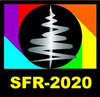#Synchrotron and Free electron laser Radiation: generation and application (SFR-2020)

13-17 July 2020
Zoom
Asia/Novosibirsk timezone

## LUMINESCENT SPECTROSCOPY OF Pr3+ IONS IN SOME PHOSPHATES, BORATES AND SILICATES USING X-RAY SYNCHROTRON RADIATION FROM VEPP-3 STORAGE RING

15 Jul 2020, 17:10
20m
Zoom 860 5034 1820 (Zoom)

### Zoom 860 5034 1820

#### Zoom

Oral X-ray spectroscopy

### Speaker

Sviatoslav Kiselev (Ural Federal University)

### Description

LUMINESCENT SPECTROSCOPY OF Pr${}^{3+}$ IONS IN SOME PHOSPHATES, BORATES AND SILICATES USING X-RAY SYNCHROTRON RADIATION FROM VEPP-3 STORAGE RING

Kiselev S.A.${}^{1,\ a)}$, Pustovarov V.A.${}^{1,\ b)}$ and Bettinelli M.${}^{2,\ c}$${}^{)} {}^{1}Institute of Physics and Technology, Ural Federal University, 19 Mira st., Ekaterinburg, 620002, Russia {}^{2\ }Laboratory of Luminescent Materials, Department of Biotechnology, University of Verona and INSTM, UdR Verona, Strada Le Grazie 15, I-37134 Verona, Italy {}^{a)}Corresponding author: sviat-kiselev@yandex.ru {}^{b)}v.a.pustovarov@urfu.ru {}^{c}$${}^{)}$ marco.bettinelli@univr.it

This paper reports the spectroscopic properties of praseodymium-doped phosphates, borates and silicates. Spectra of X-ray excited luminescence and its decay kinetics were measured. Studied samples demonstrate interconfigurational 5$d$-4$f$, intraconfigurational 4$f$-4$f$ transitions and defect-related luminescence. Comparative analysis of spectroscopic properties and application perspectives is presented.

Inorganic scintillating materials are being actively investigated in terms of their application in fields of nuclear physics, medicine tomography and detecting systems. Ce${}^{3+}$ ions impurity are widely used, but praseodymium ions are able to shift the emission spectra of interconfigurational transitions to higher energy region. The Pr${}^{3+}$ 5$d$-4$f$ emission appears, when a sufficiently strong crystal field shifts the lowest 4$f$${}^{1}5d$${}^{1}$ excited state below the ${}^{1}$S${}_{0}$ level 1.

Polycrystalline samples KLuP${}_{2}$O${}_{7}$, Li${}_{6}$Y(BO${}_{3}$)${}_{3}$, LiY${}_{6}$O${}_{5}$(BO${}_{3}$)${}_{3}$, LiSrPO${}_{4}$, Sr${}_{9}$Sc(PO${}_{4}$)${}_{7}$, K${}_{3}$Lu(PO${}_{4}$)${}_{2}$, K${}_{3}$LuSi${}_{2}$O${}_{7}$ doped with Pr${}^{3+}$ ions were synthesized using a solid state reaction and XRD verified for phase purity at the Laboratory of Luminescent Materials, University of Verona (Italy).

The measurements of emission spectra and luminescence decay kinetics upon excitation with non-monochromatic X-ray synchrotron radiation (E = 3--60 keV, pulse FWHM $\mathrm{\sim}$ 1 ns, frequency $\mathrm{\sim}$ 8 MHz) were performed at the beamline #6 of the VEPP-3 storage ring at Budker Institute of Nuclear Physics (Russia). Stroboscopic method of electron-optical chronography with sub-nanosecond time resolution was used. The detection system included a SOL Instruments MS2004 monochromator equipped with a high-speed LI-602 dissector .

For example, Fig. 1, (a) demonstrate spectra of X-ray excited luminescence of some studied samples, LiSrPO${}_{4}$:Pr${}^{3+}$, K${}_{3}$LuSi${}_{2}$O${}_{7}$:Pr${}^{3+}$ and Li${}_{6}$Y(BO${}_{3}$)${}_{3}$:Pr${}^{3+}$. Two principal regions can be observed there: 220-350 nm -- region of interconfigurational 5$d$-4$f$ transitions in Pr${}^{3+}$ impurity ions, and 480-650 nm -- region of intraconfiguration 4$f$-4$f$ transition. Decay kinetics of interconfigurational radiation transitions are presented in Fig. 2, (b). Each of them can be approximated with multiexponential description I(t) = $\mathit{\Sigma}$(A${}_{i}$*exp(-t/$\tau$${}_{i}) + I{}_{0}. Obtained luminescence lifetime parameter \tau$${}_{i}$ is vital for understanding of luminescence dynamics.

Figure1

FIGURE 1. Spectra (a) and kinetics (b) of X-ray excited luminescence,T = 295 K. The wavelength and the main lifetime $\tau$${}_{i}$ are indicated when measuring the luminescence decay kinetics

1. A.M. Srivastava, Aspects of Pr${}^{3+}$ luminescence in solids,'' J. Lumin., vol. 169, pp. 445-449, January 2016.

2. V.A. Pustovarov, E.I. Zinin, A.L. Krymov and B.V. Shulgin, Some peculiarities of the luminescence of inorganic scintillators under excitation by high intensity synchrotron radiation,'' Rev. Sci. Instrum., vol. 63, pp. 3521, September 1992.

### Summary

This paper reports the spectroscopic properties of praseodymium-doped phosphates, borates and silicates. Spectra of X-ray excited luminescence and its decay kinetics were measured. Studied samples demonstrate interconfigurational 5d-4f, intraconfigurational 4f-4f transitions and defect-related luminescence. Comparative analysis of spectroscopic properties and application perspectives is presented.

### Primary authors

Sviatoslav Kiselev (Ural Federal University) Vladimir Pustovarov (Ural Federal University)

### Presentation Materials

 LUMINESCENT SPECTROSCOPY OF Pr3+ IONS IN SOME PHOSPHATES, BORATES AND SILICATES USING X-RAY SYNCHROTRON RADIATION FROM VEPP-3 STORAGE RING.pdf Oral talk movie